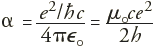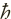Constants Topics: Values Energy Equivalents Searchable Bibliography Background Constants Bibliography Constants, Units & Uncertainty home pageIntroduction to the constants for nonexperts

 Introduction 1900 - 1920 1920 - 1940 1940 - 1960 Current (index)

Current advances: The fine-structure constant and quantum Hall effect

The fine-structure constant α is of dimension 1 (i.e., it is simply a number) and very nearly equal to 1/137. It is the "coupling constant" or measure of the strength of the electromagnetic force that governs how electrically charged elementary particles (e.g., electron, muon) and light (photons) interact. Currently, the value of α having the smallest uncertainty comes from the comparison of the theoretical expression ae(theor) and experimental value ae(expt) of the anomalous magnetic moment of the electron ae. Starting in the 1980's, a new and wholly different measurement approach using the quantum Hall effect (QHE) has caused excitement because the value of α obtained from it independently corroborates the value of α from the electron magnetic moment anomaly. The QHE value of α does not have as small an uncertainty as the electron magnetic moment value, but it does provide a significant independent confirmation of that value.

The quantity α was introduced into physics by A. Sommerfeld in 1916 and in the past has often been referred to as the Sommerfeld fine-structure constant. In order to explain the observed splitting or fine structure of the energy levels of the hydrogen atom, Sommerfeld extended the Bohr theory to include elliptical orbits and the relativistic dependence of mass on velocity. The quantity α, which is equal to the ratio v1/c where v1 is the velocity of the electron in the first circular Bohr orbit and c is the speed of light in vacuum, appeared naturally in Sommerfeld's analysis and determined the size of the splitting or fine-structure of the hydrogenic spectral lines. Sommerfeld's theory had some early success in explaining experimental observations but could not accommodate the discovery of electron spin. Although the Dirac relativistic theory of the electron introduced in 1928 solves the main aspects of the problem of the hydrogen fine-structure, α still determines its size as in the Sommerfeld theory. Consequently, the name "fine-structure" constant for the group of constants below has remained:,where e is the elementary charge,= h/2π where h is the Planck constant, ε0 = 1/µ0c2 is the electric constant (permitivity of vacuum) and µ0 is the magnetic constant (permeability of vacuum). In the International System of Units (SI), c, ε0, and µ0 are exactly known constants. Our view of the fine-structure constant has changed markedly since Sommerfeld introduced it over 80 years ago. We now consider α the coupling constant for the electromagnetic force and similar to those for the other three known fundamental forces or interactions of nature: the gravitational force, the weak nuclear force, and the strong nuclear force. Further, since α is proportional to e2, it is viewed as the square of an effective charge "screened by vacuum polarization and seen from an infinite distance." According to quantum electrodynamics (QED), the relativistic quantum field theory of the interaction of charged particles and photons, an electron can emit virtual photons that can then emit virtual electron-positron pairs (e+, e-). The virtual positrons are attracted to the original or "bare" electron while the virtual electrons are repelled from it. The bare electron is therefore screened due to this polarization. The usual fine-structure constant α is defined as the square of the completely screened charge, that is, the value observed at infinite distance or in the limit of zero momentum transfer. At shorter distances corresponding to higher energy processes or probes (large momentum transfers), the screen is partially penetrated and the strength of the electromagnetic interaction increases since the effective charge increases. Thus α depends upon the energy at which it is measured, increasing with increasing energy, and is considered an effective or running coupling constant. Indeed, due to e+ e- and other vacuum polarization processes, at an energy corresponding to the mass of the W boson (approximately 81 GeV, equivalent to a distance of approximately 2 x 10-17 m), α(mW) is approximately 1/128 compared with its zero-energy value of approximately 1/137. Thus the famous number 1/137 is not unique or especially fundamental. As indicated above, the value of alpha from the quantum Hall effect (QHE) has corroborated the value from the electron magnetic moment anomaly ae. The QHE is characteristic of a completely quantized two-dimensional electron gas. Such a gas may be realized in a high-mobility semiconductor device such as a silicon metal-oxide-semiconductor field-effect transistor (MOSFET) or GaAsAlxGa1-x As heterojunction of standard Hall bar geometry in an applied magnetic flux density B of the order of 10 T and cooled to about 1 K. For a fixed current I (typically 10 µA to 50 µA) through the device, there are regions in the curve of Hall voltage UH versus gate voltage for a MOSFET, or of UH vs B for a heterojunction, where UH remains constant as either the gate voltage or B is varied. These regions of constant UH are termed quantum Hall plateaus. In the limit of zero dissipation (zero voltage drop) in the direction of current flow, the Hall voltage-to-current quotient UH(i)/I or Hall resistance RH(i) of the ith plateau, where i is an integer (we consider only the integral QHE), is quantized and given by RH(i) = UH(i)/I = RK/i where RK is the von Klitzing constant (after the discoverer of the QHE). The theory of the QHE predicts, and the experimentally observed universality of RH(i) = UH(i)/I = RK/i is consistent with the prediction, that RK = h/e2= µ0c/2α. Since in the SI µ0 = 4π x 10-7 N/A2 exactly, and c = 299 792 458 m/s exactly as a result of the 1983 redefinition of the meter in terms of the speed of light, a measurement of RK in SI units (i.e., ohms) with a given uncertainty will yield a value of the fine structure constant α with the same uncertainty. In practice, RK is measured in terms of a laboratory standard of resistance. Thus, the resistance of the standard must be determined in the SI unit ohm in a separate experiment using an apparatus known as a calculable cross capacitor in which the unknown resistance of a reference resistor is compared with the known impedance of the capacitor. The change in capacitance of such a capacitor, and hence its change in impedance, can be readily calculated since the change depends only on the position of a movable screen electrode whose displacement can be measured with a laser interferometer. In the NIST version of the experiment, the known 0.5 pF change in capacitance of the NIST calculable cross capacitor is used to measure the capacitances of 10 pF reference capacitors. These and a 10:1 bridge are then used in two stages to measure the capacitance of two 1000 pF capacitors, which are in turn used as two arms of a special frequency dependent bridge to measure the impedances of two 100 kiloohm resistors. The latter are then compared using a 100:1 bridge with a 1000 ohm transportable resistor, which in turn is compared using dc techniques with the resistance standard in terms of which RK has been measured. The ac-dc resistance difference of the 1000 ohm resistor is determined by means of a special 1000 ohm coaxial resistor of negligible ac-dc resistance difference. All ac measurements are carried out at a frequency of approximately 1592 Hz (2πf = 104 rad/s). The QHE has already yielded a value of α with a relative standard uncertainty of 24 x 10-9. When used to compare ae(theor) with ae(expt), it gives a fractional difference of (29 ± 24) x 10-9. Since the 29 x 10-9 fractional difference is only 1.2 times the 24 x 10-9 relative standard uncertainty of the difference, it is within statistically acceptable limits. Return to Background information related to the constants.